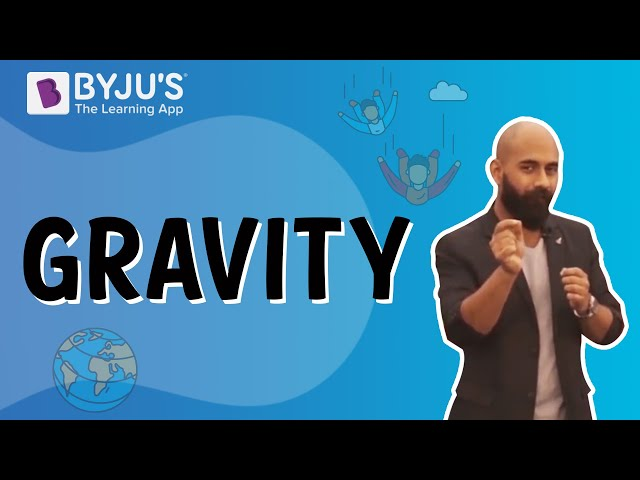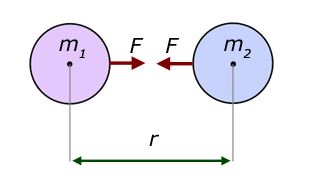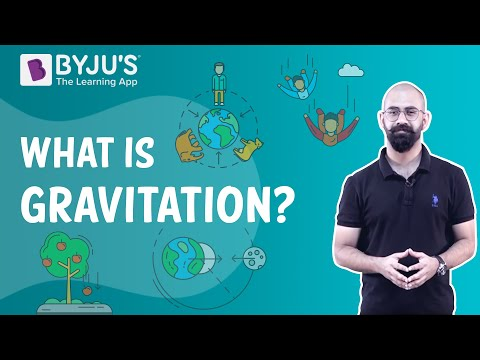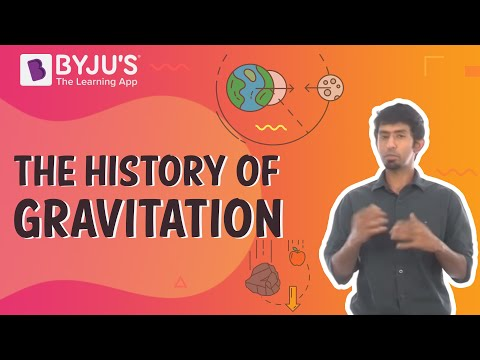# Gravitation Class 11 Physics Notes

According to the CBSE Syllabus 2023-24, this chapter has been renumbered as Chapter 7.

## What is Gravitation?

When we talk about gravitation or gravity, it is a naturally occurring phenomenon or a force which exists among all material objects in the universe.

Also, See  Types of Forces### What is Newton’s Law of Gravitation?Newton’s law of universal gravitation shows us that the gravitational force (F) of attraction between two substances let their masses be (m1) and (m2), separated by the distance (r)

$$\begin{array}{l}F = G m_{1}m_{2}/r^{2}\end{array}$$

Related links: Gravitational Constant and Universal Law Of Gravitation### What is Kepler’s Law of Planetary Motion?

Kepler’s laws of planetary motion state that:

(a) All planets move in elliptical orbits with the Sun at one of the focal points

(b) The radius vector drawn from the Sun to a planet sweeps out equal areas in equal time intervals. This follows from the fact that the force of gravitation on the planet is central, and hence angular momentum is conserved.

(c) The square of the orbital period of a planet is proportional to the cube of the semi-major axis of the elliptical orbit of the planet

 Also Access NCERT Solutions for Class 11 Physics Chapter 8 NCERT Exemplar for Class 11 Physics Chapter 8

### Important Questions

(a) You can shield a charge from electrical forces by putting it inside a hollow conductor. Can you shield a body from the gravitational influence of nearby matter by putting it inside a hollow sphere or by some other means?

(b) An astronaut inside a small spaceship orbiting around the Earth cannot detect gravity. If the space station orbiting around the earth has a large size, can he hope to detect gravity?

(c) If you compare the gravitational force on the earth due to the sun to that due to the moon, you would find that the Sun’s pull is greater than the moon’s pull. (you can check this yourself using the data available in the succeeding exercises).

1. Suppose there existed a planet that went around the sun twice as fast as the Earth. What would be its orbital size as compared to that of the Earth? However, the tidal effect of the moon’s pull is greater than the tidal effect of the sun. Why?
2. Choose the correct alternative:

(a) If the zero of potential energy is at infinity, the total energy of an orbiting satellite is negative of its kinetic/potential energy.

(b) The energy required to launch an orbiting satellite out of Earth’s gravitational influence is more/less than the energy required to project a stationary object at the same height (as the satellite) out of Earth’s influence.

• Gravitation Universal Law Of Gravitation Kepler’s law of Planetary Motion Acceleration Due to Gravity Law Of Gravitation

This was about CBSE Notes for Class 11 Physics Gravitation. Get more CBSE solutions and other study materials only at BYJU’S.

 Law of Gravitation Motion Of Celestial Bodies in Space

## Frequently Asked Questions on CBSE Class 11 Physics Notes Gravitation

Q1

### What is the Newton’s Law of Gravitation?

It states that any particle of matter in the universe attracts any other with force varying directly as the product of the masses and inversely as the square of the distance between them.

Q2

### What does planetary motion mean?

Three laws were devised by Johannes Kepler to define the mechanics of planetary motion.

Q3

### What is the Gravitational constant?

The universal gravitational constant relates the magnitude of the gravitational attractive force between two bodies to their masses and the distance between them.